##### Quantum Physics For Dummies, Revised EditionThe Hamiltonian represents the total energy of all the particles in a multi-particle system. You can describe that system in quantum physics terms. The following figure shows a multi-particle system where a number of particles are identified by their position (ignoring spin).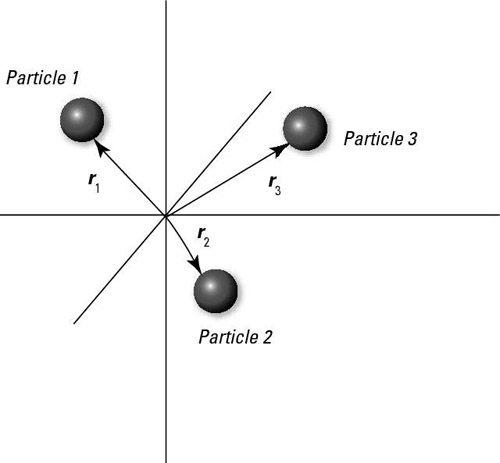To find the total energy for this system, begin by working with the wave function. The state of a system with many particles, as shown in the figure, is given by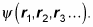And here’s the probability that particle 1 is in d3r1, particle 2 is in d3r2, particle 3 is in d3r3, and so on: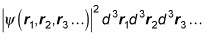The normalization of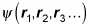demands that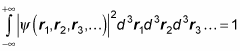Okay, so what about the Hamiltonian, which gives you the energy states? That is, what is H, where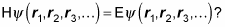When you’re dealing with a single particle, you can write this as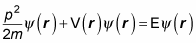But in a many-particle system the Hamiltonian must represent the total energy of all particles, not just one.

The total energy of the system is the sum of the energy of all the particles, so here’s how you can generalize the Hamiltonian for multi-particle systems without spin: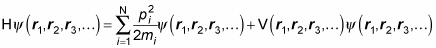This, in turn, equals the following: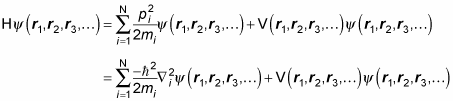Here, mi is the mass of the ith particle and V is the multi-particle potential.